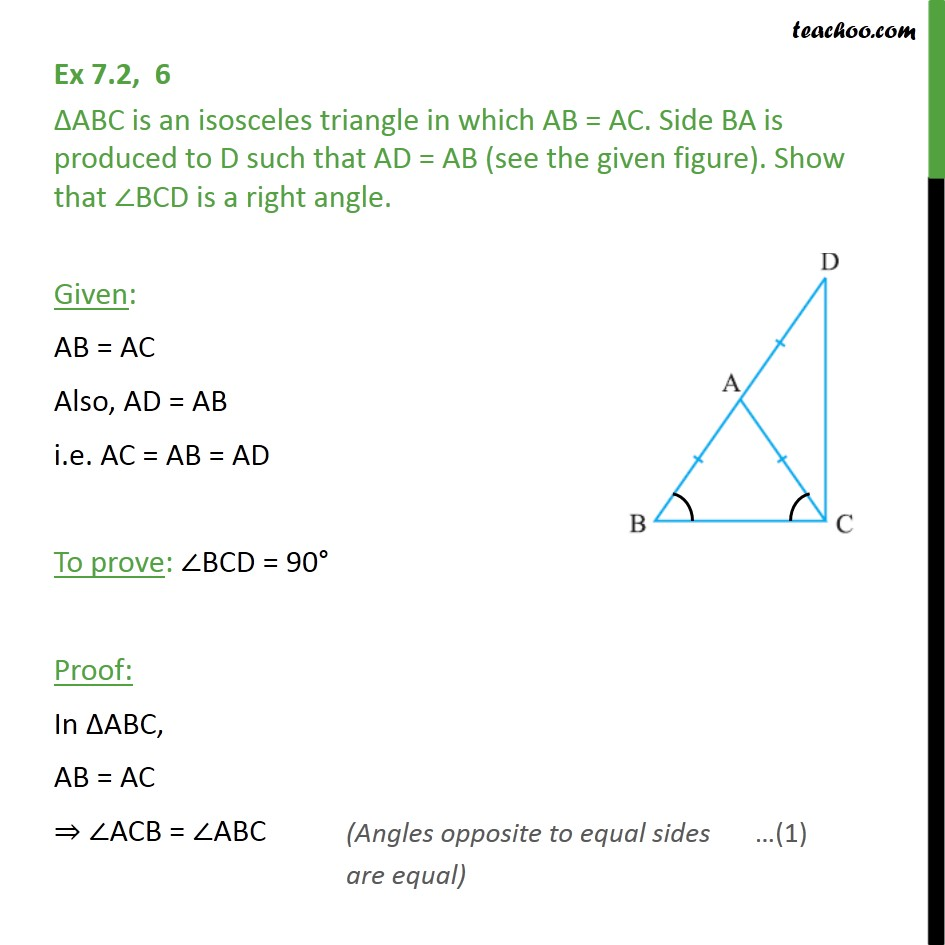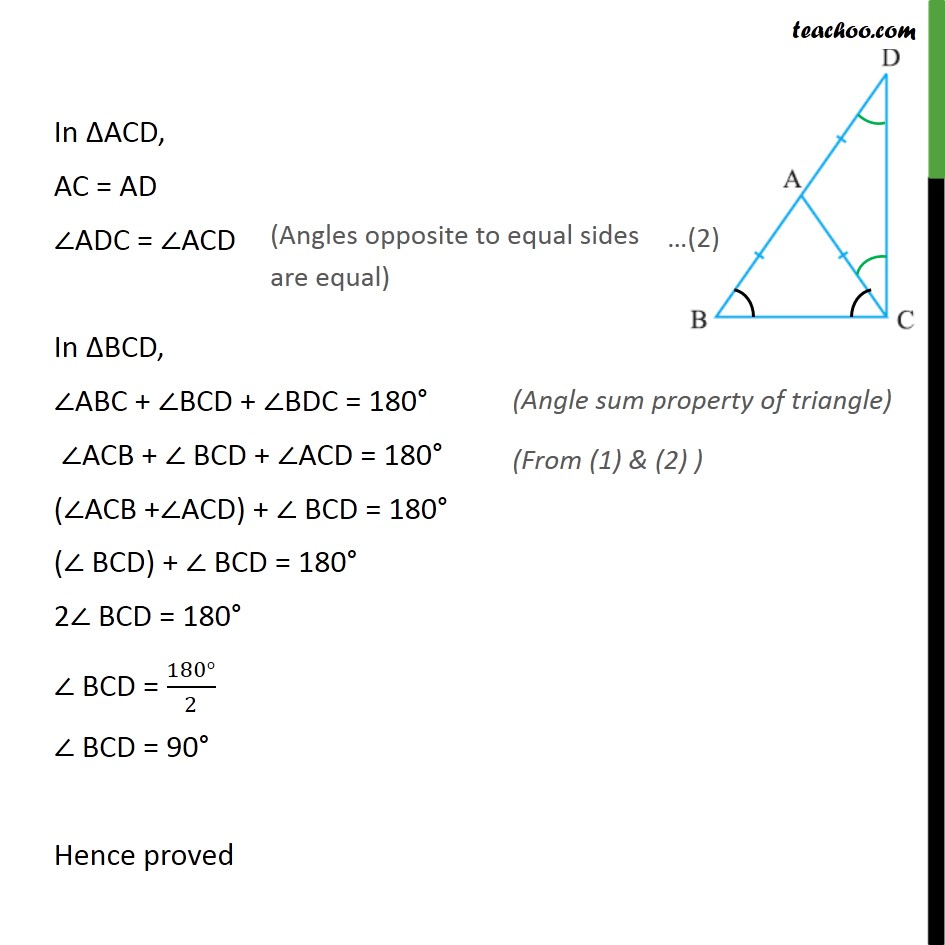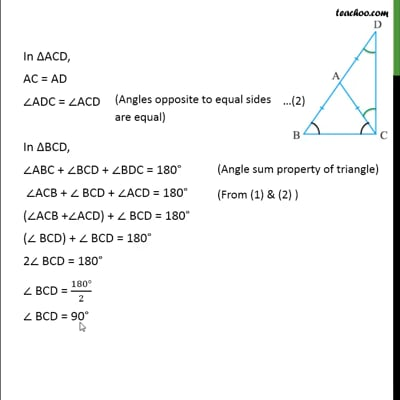Ex 7.2

Chapter 7 Class 9 Triangles
Serial order wiseThis video is only available for Teachoo black users

Maths Crash Course - Live lectures + all videos + Real time Doubt solving!

### Transcript

Ex 7.2, 6 ΔABC is an isosceles triangle in which AB = AC. Side BA is produced to D such that AD = AB (see the given figure). Show that ∠BCD is a right angle. Given: AB = AC Also, AD = AB i.e. AC = AB = AD To prove: ∠BCD = 90° Proof: In ΔABC, AB = AC ⇒ ∠ACB = ∠ABC In ΔACD, AC = AD ∠ADC = ∠ACD In ΔBCD, ∠ABC + ∠BCD + ∠BDC = 180° ∠ACB + ∠ BCD + ∠ACD = 180° (∠ACB +∠ACD) + ∠ BCD = 180° (∠ BCD) + ∠ BCD = 180° 2∠ BCD = 180° ∠ BCD = (180°)/2 ∠ BCD = 90° Hence proved